# Liquid Solutions

## 30 Questions MCQ Test | Liquid Solutions

Description
Attempt Liquid Solutions | 30 questions in 45 minutes | Mock test for preparation | Free important questions MCQ to study for Exam | Download free PDF with solutions
QUESTION: 1

Solution:
QUESTION: 2

Solution:
QUESTION: 3

### .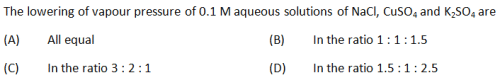Solution:
QUESTION: 4
.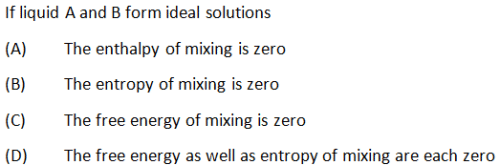Solution:
QUESTION: 5
.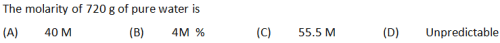Solution:
QUESTION: 6
.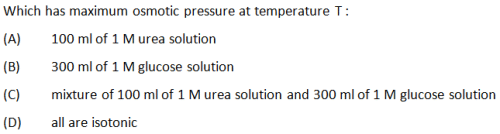Solution:
QUESTION: 7

The addition of a small amount of ___________ to H2O may actually reduce friction compared to H2O alone.

Solution:

The addition should be done on the order of 0.3% consistency although it can be higher at higher flow velocities, so it could actually reduce friction compared to H2O alone.

QUESTION: 8
.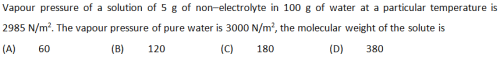Solution:
QUESTION: 9

The elevation in the boiling point for 13.44 g of CuCl2 dissolved in 1Kg of water as solvent will be (Kb = 0.52 k kg mol-1 ; molar mass of CuCl2 = 134.4 g/mol )

Solution:

Explanation : ΔTb = iKfm

ΔTb = [1000 × 0.52 × 13.44(1+2α)]/[134.4 × 1000]

ΔTb = 0.156 (∵α = 1)

QUESTION: 10
.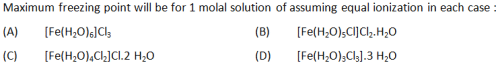Solution:
QUESTION: 11
.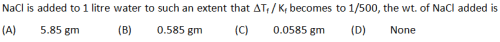Solution:
QUESTION: 12
.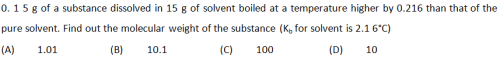Solution:
QUESTION: 13
.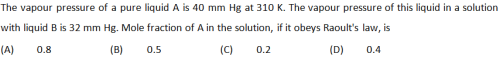Solution:
QUESTION: 14
.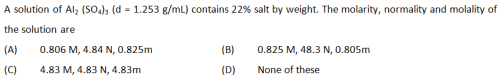Solution:
QUESTION: 15
.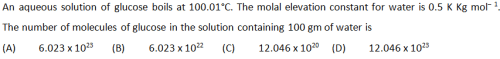Solution:
QUESTION: 16
.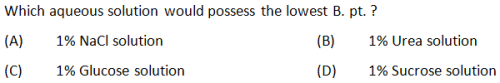Solution:
QUESTION: 17
.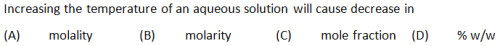Solution:
QUESTION: 18
.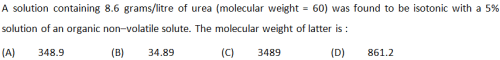Solution:
QUESTION: 19
.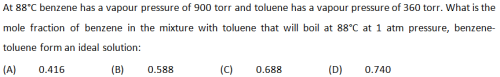Solution:
QUESTION: 20
.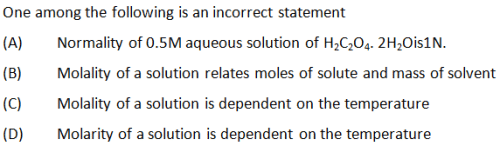Solution:
QUESTION: 21
.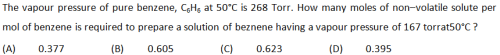Solution:
QUESTION: 22
.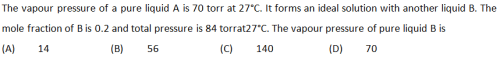Solution:
QUESTION: 23
.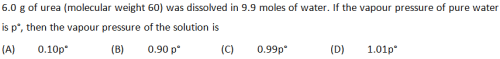Solution:
QUESTION: 24
.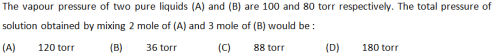Solution:
QUESTION: 25
.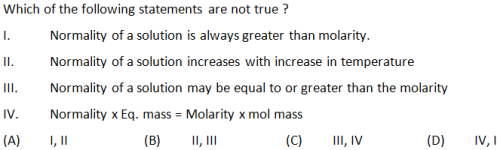Solution:
QUESTION: 26
.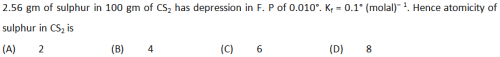Solution:
QUESTION: 27
.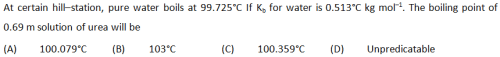Solution:
QUESTION: 28
.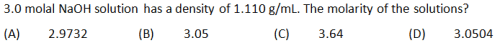Solution:
QUESTION: 29
.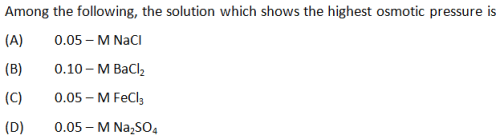Solution:
QUESTION: 30
.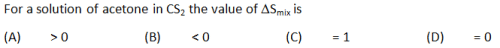Solution:Use Code STAYHOME200 and get INR 200 additional OFF Use Coupon Code